## 1. Introduction

Project objective:
Our exploration will enable us to identify taxi trip patterns in NYC and develop strategies accordingly to improve revenue for taxi drivers and taxi companies.

We combined datasets from 2018 to 2021 to draw more insight from our analysis. As data from 2020 onwards show the impact of the pandemic on taxi demand, providing information from the two years prior to the pandemic would lead to a more accurate interpretation of results.

For Phase 1 of our project we explored the data to understand commonalities in demand, depending on year, month, day of the week, time of the day and location. In Phase 2 we utilized our findings to discern further how those factors affect revenue.

Motivation:
Taxi market has been facing great competition in recent years, as an increasing number of people switch from taxi to share-riding, such as Uber and Lyft, as a means of transportation. While taxi companies and drivers may have a hard time going through this transition, there are people who prefer and need to take taxis. By analyzing taxi trip patterns in NYC, we will help taxi companies and drivers learn more about the customers they're serving and, more importantly, how to increase revenue to stay in business.

The analysis revealed that taxi trips were most popular during the morning and afternoon hours. Short-distance trips were the most popular, with the most frequently traveled routes being in the upper east and upper west side, spanning 66 blocks. Long trips were found to be the most expensive per minute. In terms of difference in demand based on the day of the week; Friday has the highest demand, while Sunday has the lowest.

Report Summary:
We cleaned the dataframe to exclude unrepresentative data points and created features that better fit the purpose of our analyses. We conducted exploratory data analysis on taxi trip demand patterns, revenue patterns, and how they interrelate to one another. We also implemented machine learning methods, including linear regression, random forest, and GBT regression, to predict taxi trip price based on other features.

## 2. Data source

Data Source:
The datasets used were downloaded from BigQuery. The information in this dataset was made available by the New York City Taxi and Limousine Commission (TLC).

This project used datasets containing data regarding yellow taxi trips in New York City spanning from 2018 to 2021. We also used a taxi zone dataset to assign name locations to the zone_ids, which by itself, would not sufficiently contextualize the data.

We decided not to include data from 2022 as early exploration of that dataset indicated that values from the month of December were missing from the original dataset featured on BigQuery.

Data dictionary
taxi_zone_geom

bigquery-public-data.new_york_taxi_trips.taxi_zone_geom

Column Name Description Type
zone_id Unique ID number of each taxi zone. Corresponds with the pickup_location_id and dropoff_location_id in each of the trips tables STRING
zone_name Full text name of the taxi zone STRING
borough Borough containing the taxi zone STRING
zone_geom Geometric outline that defines the taxi zone suitable for GIS analysis. GEOGRAPHY

tlc_yellow_trips

2018: bigquery-public-data.new_york_taxi_trips.tlc_yellow_trips_2018

2019: bigquery-public-data.new_york_taxi_trips.tlc_yellow_trips_2019

2020: bigquery-public-data.new_york_taxi_trips.tlc_yellow_trips_2020

2021: bigquery-public-data.new_york_taxi_trips.tlc_yellow_trips_2021

Column Name Description Type
vendor_id A code indicating the LPEP provider that provided the record. 1= Creative Mobile Technologies, LLC; 2= VeriFone Inc. STRING
pickup_datetime The date and time when the meter was engaged TIMESTAMP
dropoff_datetime The date and time when the meter was disengaged TIMESTAMP
passenger_count The number of passengers in the vehicle. This is a driver-entered value. INTEGER
trip_distance The elapsed trip distance in miles reported by the taximeter. NUMERIC
rate_code The final rate code in effect at the end of the trip. 1= Standard rate 2=JFK 3=Newark 4=Nassau or Westchester 5=Negotiated fare 6=Group ride STRING
store_and_fwd_flag This flag indicates whether the trip record was held in vehicle memory before sending to the vendor, aka 'store and forward,' because the vehicle did not have a connection to the server. Y= store and forward trip N= not a store and forward trip STRING
payment_type A numeric code signifying how the passenger paid for the trip. 1= Credit card 2= Cash 3= No charge 4= Dispute 5= Unknown 6= Voided trip STRING
fare_amount The time-and-distance fare calculated by the meter NUMERIC
extra Miscellaneous extras and surcharges. Currently, this only includes the 0.50 and 1 dollar rush hour and overnight charges NUMERIC
mta_tax 0.50 dollar MTA tax that is automatically triggered based on the metered rate in use NUMERIC
tip_amount Tip amount. This field is automatically populated for credit card tips. Cash tips are not included. NUMERIC
tolls_amount Total amount of all tolls paid in trip. NUMERIC
imp_surcharge 0.30 dollar improvement surcharge assessed on hailed trips at the flag drop. The improvement surcharge began being levied in 2015. NUMERIC
airport_fee - NUMERIC
total_amount The total amount charged to passengers. Does not include cash tips. NUMERIC
pickup_location_id TLC Taxi Zone in which the taximeter was engaged STRING
dropoff_location_id TLC Taxi Zone in which the taximeter was disengaged STRING
data_file_year Datafile timestamp year value INTEGER
data_file_month Datafile timestamp month value INTEGER

## 3. Data Cleaning

```data2021 = spark.read.format('bigquery').option('table', 'bigquery-public-data:new_york_taxi_trips.tlc_yellow_trips_2021').load()
df_raw = data2021.union(data2020).union(data2019).union(data2018)
df_raw.printSchema()
df_raw.show(5)
```
```df_raw = data2021.union(data2020).union(data2019).union(data2018)
df_raw.printSchema()
df_raw.show(5)
```
```root
|-- vendor_id: string (nullable = false)
|-- pickup_datetime: timestamp (nullable = true)
|-- dropoff_datetime: timestamp (nullable = true)
|-- passenger_count: long (nullable = true)
|-- trip_distance: decimal(38,9) (nullable = true)
|-- rate_code: string (nullable = true)
|-- store_and_fwd_flag: string (nullable = true)
|-- payment_type: string (nullable = true)
|-- fare_amount: decimal(38,9) (nullable = true)
|-- extra: decimal(38,9) (nullable = true)
|-- mta_tax: decimal(38,9) (nullable = true)
|-- tip_amount: decimal(38,9) (nullable = true)
|-- tolls_amount: decimal(38,9) (nullable = true)
|-- imp_surcharge: decimal(38,9) (nullable = true)
|-- airport_fee: decimal(38,9) (nullable = true)
|-- total_amount: decimal(38,9) (nullable = true)
|-- pickup_location_id: string (nullable = true)
|-- dropoff_location_id: string (nullable = true)
|-- data_file_year: long (nullable = true)
|-- data_file_month: long (nullable = true)

```
```[Stage 0:>                                                          (0 + 1) / 1]
```
```+---------+-------------------+-------------------+---------------+-------------+---------+------------------+------------+------------+-----------+-----------+-----------+------------+-------------+-----------+------------+------------------+-------------------+--------------+---------------+
|vendor_id|    pickup_datetime|   dropoff_datetime|passenger_count|trip_distance|rate_code|store_and_fwd_flag|payment_type| fare_amount|      extra|    mta_tax| tip_amount|tolls_amount|imp_surcharge|airport_fee|total_amount|pickup_location_id|dropoff_location_id|data_file_year|data_file_month|
+---------+-------------------+-------------------+---------------+-------------+---------+------------------+------------+------------+-----------+-----------+-----------+------------+-------------+-----------+------------+------------------+-------------------+--------------+---------------+
|        1|2021-01-01 00:43:30|2021-01-01 01:11:06|              1| 14.700000000|      1.0|                 N|           1|42.000000000|0.500000000|0.500000000|8.650000000|        0E-9|  0.300000000|       null|51.950000000|               132|                165|          2021|              1|
|        1|2021-01-01 00:15:48|2021-01-01 00:31:01|              0| 10.600000000|      1.0|                 N|           1|29.000000000|0.500000000|0.500000000|6.050000000|        0E-9|  0.300000000|       null|36.350000000|               138|                132|          2021|              1|
|        2|2021-01-01 00:19:57|2021-01-01 00:43:03|              3| 10.740000000|      1.0|                 N|           1|32.500000000|0.500000000|0.500000000|4.000000000|        0E-9|  0.300000000|       null|40.300000000|               264|                231|          2021|              1|
|        2|2021-01-01 00:44:58|2021-01-01 01:07:41|              2|  5.850000000|      1.0|                 N|           1|21.500000000|0.500000000|0.500000000|5.060000000|        0E-9|  0.300000000|       null|30.360000000|               249|                238|          2021|              1|
|        2|2021-01-01 00:06:11|2021-01-01 00:23:40|              1|  8.060000000|      1.0|                 N|           1|24.500000000|0.500000000|0.500000000|5.660000000|        0E-9|  0.300000000|       null|33.960000000|                75|                264|          2021|              1|
+---------+-------------------+-------------------+---------------+-------------+---------+------------------+------------+------------+-----------+-----------+-----------+------------+-------------+-----------+------------+------------------+-------------------+--------------+---------------+
only showing top 5 rows

```
```
```

### 3.b. Dropping Unnecessary Columns

The columns 'data_file_year' and 'data_file_month' are not of interest, so we drop them here.

```df_raw = df_raw.drop('data_file_year','data_file_month')
```
```df_raw.cache()
```
`DataFrame[vendor_id: string, pickup_datetime: timestamp, dropoff_datetime: timestamp, passenger_count: bigint, trip_distance: decimal(38,9), rate_code: string, store_and_fwd_flag: string, payment_type: string, fare_amount: decimal(38,9), extra: decimal(38,9), mta_tax: decimal(38,9), tip_amount: decimal(38,9), tolls_amount: decimal(38,9), imp_surcharge: decimal(38,9), airport_fee: decimal(38,9), total_amount: decimal(38,9), pickup_location_id: string, dropoff_location_id: string]`

### 3.c. Handling missing values

Here we sample a portion from the whole dataframe only for more efficient visualization of missing values. We'll conduct the actually data cleaning steps on the original dataframe based on the pattern observed from the sample. The sample is 0.05% of the entire dataframe, giving us over 0.1 million records. This sample is chosen to be this size for the purpose of efficient code execution.

```df_raw_sample = df_raw.sample(False, 0.0005, 843)
df_raw_sample.cache()
```
`DataFrame[vendor_id: string, pickup_datetime: timestamp, dropoff_datetime: timestamp, passenger_count: bigint, trip_distance: decimal(38,9), rate_code: string, store_and_fwd_flag: string, payment_type: string, fare_amount: decimal(38,9), extra: decimal(38,9), mta_tax: decimal(38,9), tip_amount: decimal(38,9), tolls_amount: decimal(38,9), imp_surcharge: decimal(38,9), airport_fee: decimal(38,9), total_amount: decimal(38,9), pickup_location_id: string, dropoff_location_id: string]`
```import matplotlib.pyplot as plt
import seaborn as sns
```

Upon investigation, we find four columns with missing values, which are not missing at random. The observations of columns "passenger_count", and "rate_code" are missing only when "payment_type" is 0 and vice versa. Moreover, payment_type that has a value of 0 is not described in the data dictionary, so we will drop these rows.

```df_raw_sample = df_raw_sample.where(~(df_raw_sample['payment_type']==0))
df_raw = df_raw.where(~(df_raw_sample['payment_type']==0))
```

The airport_fee column had no values until a the end of March in 2021. We fill the missing values of "airport_fee" with -999 so we can keep the column for further analysis.

```df_raw = df_raw.fillna(-999,subset=['airport_fee'])
```
```df_raw.cache()
```
`DataFrame[vendor_id: string, pickup_datetime: timestamp, dropoff_datetime: timestamp, passenger_count: bigint, trip_distance: decimal(38,9), rate_code: string, store_and_fwd_flag: string, payment_type: string, fare_amount: decimal(38,9), extra: decimal(38,9), mta_tax: decimal(38,9), tip_amount: decimal(38,9), tolls_amount: decimal(38,9), imp_surcharge: decimal(38,9), airport_fee: decimal(38,9), total_amount: decimal(38,9), pickup_location_id: string, dropoff_location_id: string]`

### 3.d.1. Handling outliers based on time and trip duration

```from pyspark.sql.functions import year, hour, unix_timestamp, col, round
# Extract year as a column
df_raw = df_raw.withColumn('year',year(df_raw['pickup_datetime']))
```

As our dataset should only contain data from 2018 to 2021, we therefore drop all rows that are not within this year range.

```df_raw = df_raw.where((df_raw['year']==2021)|(df_raw['year']==2020)|(df_raw['year']==2019)|(df_raw['year']==2018))
```

Drop rows where pickup time is no earlier than dropoff time.

```df_raw = df_raw.where(df_raw['pickup_datetime']<df_raw['dropoff_datetime'])
```

In the following step, we're handling outliers based on the common sense that most taxi trips wouldn't take extremely long hours. We'll calculate the duration in minutes for each taxi trip and eliminate data points that fall out of the range of mean +/- 2.5 standard deviations, as is often the convention in statistical analysis.

```df_raw = df_raw.withColumn("duration", (unix_timestamp(col("dropoff_datetime")) - unix_timestamp(col("pickup_datetime"))) / 60)
```
```df_raw = df_raw.withColumn("duration", round(col("duration"), 2))
```
```std_duration = df_raw.agg({'duration': 'stddev'}).collect()
mean_duration = df_raw.agg({'duration': 'mean'}).collect()
hi_bound_duration = mean_duration + (2.5 * std_duration)
low_bound_duration = mean_duration - (2.5 * std_duration)
df_raw = df_raw.where((df_raw['duration']>low_bound_duration)&(df_raw['duration']<hi_bound_duration))
```
```
```
```df_raw.cache()
```
`DataFrame[vendor_id: string, pickup_datetime: timestamp, dropoff_datetime: timestamp, passenger_count: bigint, trip_distance: decimal(38,9), rate_code: string, store_and_fwd_flag: string, payment_type: string, fare_amount: decimal(38,9), extra: decimal(38,9), mta_tax: decimal(38,9), tip_amount: decimal(38,9), tolls_amount: decimal(38,9), imp_surcharge: decimal(38,9), airport_fee: decimal(38,9), total_amount: decimal(38,9), pickup_location_id: string, dropoff_location_id: string, year: int, duration: double]`

### 3.d.2. Handling outliers based on price and rate code

Each taxi trip is priced according to a fixed set of rules, where the major component of pricing is attributed to distance-and-time fare calculated by the meter (in our dataset, the "fare_amount" variable). While other fees and surcharges also apply to some taxi trips, they only account for a much smaller portion of taxi pricing. Based on this knowledge, we will exliminate "abnormal" data points that don't follow such patterns.

We noticed there exist out-of-range values (the value "99.0", which is not a valid rate_code according to the data dictionary) and abnormal values (e.g. "1.0" and "1" exist at the same time) for the rate_code column, so we fix them here using the following code:

```df_raw = df_raw.where((df_raw['rate_code'] != '99.0')&(df_raw['rate_code'] != '99'))

from pyspark.sql.functions import when
df_raw = df_raw.withColumn('rate_code', when(df_raw['rate_code']=='1.0','1')\
.when(df_raw['rate_code']=='2.0','2')\
.when(df_raw['rate_code']=='3.0','3')\
.when(df_raw['rate_code']=='4.0','4')\
.when(df_raw['rate_code']=='5.0','5')\
.when(df_raw['rate_code']=='6.0','6')
.otherwise(df_raw['rate_code']))
```
```df_raw.select(col('rate_code')).distinct().show()
```
```
```
```+---------+
|rate_code|
+---------+
|        3|
|        5|
|        6|
|        1|
|        4|
|        2|
+---------+

```
```
```

In the next step, we create two plots describing the correlation between trip distance and distance-and-time calculated fare. The plot on the left-hand side is for all trips, including standard-rate trips, airport trips, and "negotiated price" trips. The plot on the right-hand side only includes standard-rate trips whose fare is calculated by the meter based on distance and time.

• When rate_code equals 1, the corresponding row represents a trip charging standard rate, meaning that the fare_amount column reflects a fare calculated based on distance and time. The base fare for such trips from 2018 to 2021 is \\$2.5. Each additional mile charges another \\\$2.5 and each additional minute charges another \\$0.5. Therefore, the correlation between trip distance and fare_amount should be linear with a slope equal to or above 2.5.
• When rate_code is 2 or 3 or 4, the corresponding row represents an airport taxi trip that uses a different base fare from standard rate. For example, there is a flat rate of \\$52 for trips between Manhattan to JFK airport, and such trips have a rate_code of 2 in the dataset.
• When rate_code equals 5 or 6, the row represents a taxi trip with a negotiated price whose trip distance does not follow a linear correlation with fare_amount.

As can be seen from the plots below, there are data points that do not follow the above rules and should be fixed.

```df_raw_sample = df_raw.sample(False,0.0005,843)
df_pd = df_raw_sample.toPandas()
```
```
```
```df_pd['trip_distance']= df_pd['trip_distance'].astype('float')
df_pd['fare_amount']= df_pd['fare_amount'].astype('float')
df_pd['total_amount']= df_pd['total_amount'].astype('float')
df_pd['tip_amount']= df_pd['tip_amount'].astype('float')
df_pd['extra']= df_pd['extra'].astype('float')
df_pd['mta_tax']= df_pd['mta_tax'].astype('float')
df_pd['tolls_amount']= df_pd['tolls_amount'].astype('float')
df_pd['imp_surcharge']= df_pd['imp_surcharge'].astype('float')
df_pd['airport_fee']= df_pd['airport_fee'].astype('float')
```
```fig, ax = plt.subplots(1, 2, figsize=(12, 6))

sns.scatterplot(x='trip_distance',y='fare_amount',data=df_pd,hue='rate_code',ax=ax)
ax.set_title("Distance-fare Correlation for Standard Rate Trips")
ax.legend(loc='lower right',title='rate_code')

sns.scatterplot(x='trip_distance',y='fare_amount',data=df_pd[df_pd['rate_code']=='1'],ax=ax,hue='rate_code')
ax.set_title("Distance-fare Correlation for Standard Rate Trips")
ax.legend(loc='lower right',title='rate_code')

plt.show()
```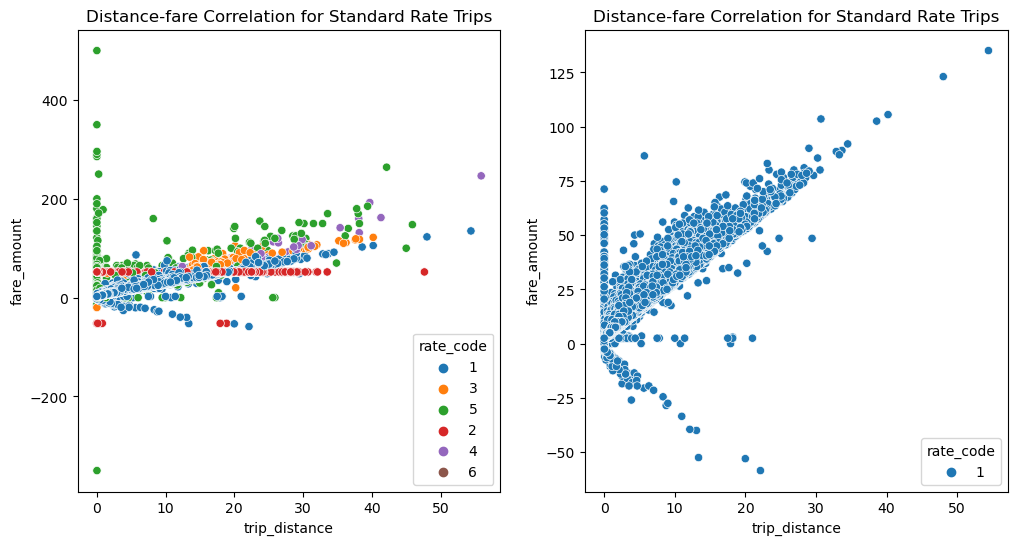We use the code below to fix the data:

• For all trips, we eliminate records that have a 0 or negative trip distance.
• For all trips, we eliminate records with a total_amount less than \\$2.5.
• For standard rate trips, we eliminate records whose trip distance doesn't folllow a linear correlation with a slope of at least 2.5 with fare_amount.
• For non-standard-rate trips, because the correlation between trip distance and fare_amount largely depend on actual negotiation between customers and drivers, we will keep those data points where trip distance and fare_amount don't follow a linear correlation as discussed before.
```df_standard_rate =df_raw.where((df_raw['rate_code']=='1')&(df_raw['trip_distance']>0)&(df_raw['total_amount']>=2.5)&(df_raw['fare_amount']/df_raw['trip_distance']>=2.5))
df_other_rates =df_raw.where((df_raw['rate_code']!='1')&(df_raw['trip_distance']>0)&(df_raw['total_amount']>=2.5))
```

Dataframe, df, created below is a clean version that has addressed all missing values, outliers, and abnormal records.

```df = df_standard_rate.union(df_other_rates)
```

Below are plots that describe the correlation between trip distance and fare after outliers are eliminated.

```import pandas as pd
df_pd = pd.concat([df_standard_rate_pd,df_other_rates_pd])
```
```fig, ax = plt.subplots(1, 2, figsize=(12, 6))

sns.scatterplot(x='trip_distance',y='fare_amount',data=df_pd,hue='rate_code',hue_order = ['1', '2','3','4','5','6'],ax=ax)
ax.set_title("Distance-fare Correlation for All Trips (Clean)")
ax.legend(loc='lower right',title='rate_code')

sns.scatterplot(x='trip_distance',y='fare_amount',data=df_pd[df_pd['rate_code']=='1'],ax=ax,hue='rate_code')
ax.set_title("Distance-fare Correlation for Standard Rate Trips (Clean)")
ax.legend(loc='lower right',title='rate_code')

plt.show()
```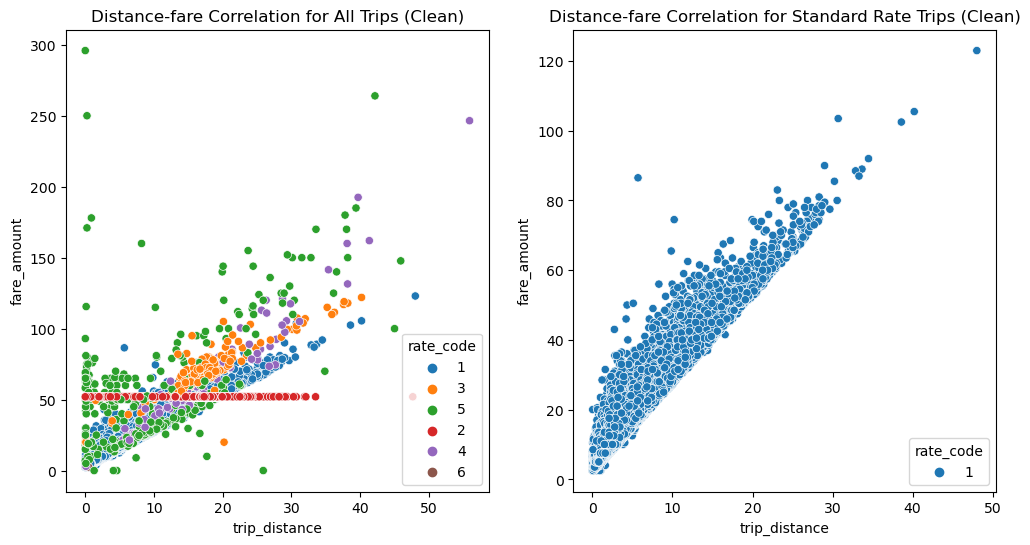The right-hand side plot suggests that the fare for JFK trips, represented by the red dots, are quite fixed no matter how long the trip actually covers. Trips other than JFK and negotiated-price trips mostly follow linear distribution between distance and fare. Negotiated-price trips seem to follow a more unusual distribution, which might depend on the actual negotiation between customers and drivers.

Here we also create the same plots for trip distance and total price (not the fare calculated merely from distance and time). The correlation reflected in the plots resemble the fare-counterpart a lot, because there're other fees, surcharges, tax, and tips involved.

```fig, ax = plt.subplots(1, 2, figsize=(12, 6))

sns.scatterplot(x='trip_distance',y='total_amount',data=df_pd,hue='rate_code',ax=ax)
ax.set_title("Distance-price Correlation for All Trips (Clean)")
ax.legend(loc='lower right',title='rate_code')

sns.scatterplot(x='trip_distance',y='total_amount',data=df_pd[df_pd['rate_code']=='1'],ax=ax,hue='rate_code')
ax.set_title("Distance-price Correlation for Standard Rate Trips (Clean)")
ax.legend(loc='lower right',title='rate_code')

plt.show()
```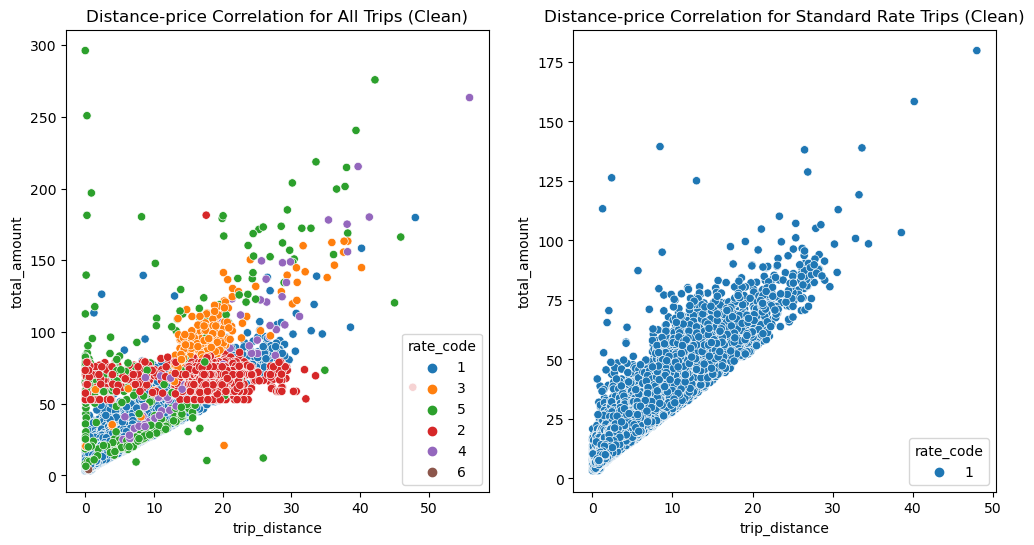### 3.e. Feature Engineering

```from pyspark.sql.types import TimestampType

EDT_start = "2021-03-14"
EST_start = "2021-11-07"
```
```from pyspark.sql.functions import from_utc_timestamp
```

Because pickup/dropoff time in the original dataset is indicated by UTC, we here convert UTC to Eastern Time

```df = df.withColumn(
"pickup_ET",
from_utc_timestamp('pickup_datetime','America/New_York')
)

df = df.withColumn(
"dropoff_ET",
from_utc_timestamp('dropoff_datetime','America/New_York')
)
```
```df.printSchema()
```
```root
|-- vendor_id: string (nullable = false)
|-- pickup_datetime: timestamp (nullable = true)
|-- dropoff_datetime: timestamp (nullable = true)
|-- passenger_count: long (nullable = true)
|-- trip_distance: decimal(38,9) (nullable = true)
|-- rate_code: string (nullable = true)
|-- store_and_fwd_flag: string (nullable = true)
|-- payment_type: string (nullable = true)
|-- fare_amount: decimal(38,9) (nullable = true)
|-- extra: decimal(38,9) (nullable = true)
|-- mta_tax: decimal(38,9) (nullable = true)
|-- tip_amount: decimal(38,9) (nullable = true)
|-- tolls_amount: decimal(38,9) (nullable = true)
|-- imp_surcharge: decimal(38,9) (nullable = true)
|-- airport_fee: decimal(38,9) (nullable = true)
|-- total_amount: decimal(38,9) (nullable = true)
|-- pickup_location_id: string (nullable = true)
|-- dropoff_location_id: string (nullable = true)
|-- year: integer (nullable = true)
|-- duration: double (nullable = true)
|-- dropoff_ET: timestamp (nullable = true)
|-- pickup_ET: timestamp (nullable = true)

```
```df.select('pickup_datetime','pickup_ET','dropoff_datetime','dropoff_ET').show(5)
```
```+-------------------+-------------------+-------------------+-------------------+
|    pickup_datetime|          pickup_ET|   dropoff_datetime|         dropoff_ET|
+-------------------+-------------------+-------------------+-------------------+
|2021-01-01 00:43:30|2020-12-31 19:43:30|2021-01-01 01:11:06|2020-12-31 20:11:06|
|2021-01-01 00:15:48|2020-12-31 19:15:48|2021-01-01 00:31:01|2020-12-31 19:31:01|
|2021-01-01 00:19:57|2020-12-31 19:19:57|2021-01-01 00:43:03|2020-12-31 19:43:03|
|2021-01-01 00:44:58|2020-12-31 19:44:58|2021-01-01 01:07:41|2020-12-31 20:07:41|
|2021-01-01 00:06:11|2020-12-31 19:06:11|2021-01-01 00:23:40|2020-12-31 19:23:40|
+-------------------+-------------------+-------------------+-------------------+
only showing top 5 rows

```

Create a column "day_of_week" to indicate the day of week for analysis

```from pyspark.sql.functions import date_format
df = df.withColumn('day_of_week', date_format('pickup_ET','EEEE'))
```

Extract the hour of the day for analysis

```df = df.withColumn('hour', hour(df['pickup_ET']))
```
```df.select('pickup_ET','hour').show(5)
```
```+-------------------+----+
|          pickup_ET|hour|
+-------------------+----+
|2020-12-31 19:43:30|  19|
|2020-12-31 19:15:48|  19|
|2020-12-31 19:19:57|  19|
|2020-12-31 19:44:58|  19|
|2020-12-31 19:06:11|  19|
+-------------------+----+
only showing top 5 rows

```
```df_raw.unpersist()
```
`DataFrame[vendor_id: string, pickup_datetime: timestamp, dropoff_datetime: timestamp, passenger_count: bigint, trip_distance: decimal(38,9), rate_code: string, store_and_fwd_flag: string, payment_type: string, fare_amount: decimal(38,9), extra: decimal(38,9), mta_tax: decimal(38,9), tip_amount: decimal(38,9), tolls_amount: decimal(38,9), imp_surcharge: decimal(38,9), airport_fee: decimal(38,9), total_amount: decimal(38,9), pickup_location_id: string, dropoff_location_id: string, year: int, duration: double, dropoff_ET: timestamp, pickup_ET: timestamp, day_of_week: string, hour: int]`
```df.cache()
```
`DataFrame[vendor_id: string, pickup_datetime: timestamp, dropoff_datetime: timestamp, passenger_count: bigint, trip_distance: decimal(38,9), rate_code: string, store_and_fwd_flag: string, payment_type: string, fare_amount: decimal(38,9), extra: decimal(38,9), mta_tax: decimal(38,9), tip_amount: decimal(38,9), tolls_amount: decimal(38,9), imp_surcharge: decimal(38,9), airport_fee: decimal(38,9), total_amount: decimal(38,9), pickup_location_id: string, dropoff_location_id: string, year: int, duration: double, pickup_ET: timestamp, dropoff_ET: timestamp, day_of_week: string, hour: int]`
```df.printSchema()
```
```root
|-- vendor_id: string (nullable = false)
|-- pickup_datetime: timestamp (nullable = true)
|-- dropoff_datetime: timestamp (nullable = true)
|-- passenger_count: long (nullable = true)
|-- trip_distance: decimal(38,9) (nullable = true)
|-- rate_code: string (nullable = true)
|-- store_and_fwd_flag: string (nullable = true)
|-- payment_type: string (nullable = true)
|-- fare_amount: decimal(38,9) (nullable = true)
|-- extra: decimal(38,9) (nullable = true)
|-- mta_tax: decimal(38,9) (nullable = true)
|-- tip_amount: decimal(38,9) (nullable = true)
|-- tolls_amount: decimal(38,9) (nullable = true)
|-- imp_surcharge: decimal(38,9) (nullable = true)
|-- airport_fee: decimal(38,9) (nullable = true)
|-- total_amount: decimal(38,9) (nullable = true)
|-- pickup_location_id: string (nullable = true)
|-- dropoff_location_id: string (nullable = true)
|-- year: integer (nullable = true)
|-- duration: double (nullable = true)
|-- dropoff_ET: timestamp (nullable = true)
|-- pickup_ET: timestamp (nullable = true)
|-- day_of_week: string (nullable = true)
|-- hour: integer (nullable = true)

```

## 4. Summary Statistics

The clean dataframe has rows and columns.

```print(df.count(),len(df.columns))
```
```[Stage 54:=====================================================>(114 + 1) / 115]
```
```239441040 24
```
```
```

### 4.a. Distribution of duration, distance, and price

The distribution of three key columns are displayed below.

• The range of taxi trip durations is from 0.02 to 153 minutes with the average being 14 minutes. There's more concentration in short-duration according to the histgram.
• The range of taxi trip distance is from 0.01 to 167,329 miles with the average being 3 miles. Most data points fall under the lower range of the distance span. (Eliminating extreme values for trip distances takes too long, so it was not done due to time ristriction)
• The range of taxi trip prices is from \\$2.5 to \\\$998,325 dollars with the average being \\$18. The majority of data points fall under the range of 2.5 to 30 dollars. (Eliminating extreme values for total price takes too long, so it was not done due to time ristriction)
```print(df.select('duration').describe().show(),df.select('trip_distance').describe().show(),df.select('total_amount').describe().show())
```
```
```
```+-------+------------------+
|summary|          duration|
+-------+------------------+
|  count|         236937680|
|   mean|14.202429541683667|
| stddev|11.379465486849186|
|    min|              0.02|
|    max|            185.52|
+-------+------------------+

```
```
```
```+-------+------------------+
|summary|     trip_distance|
+-------+------------------+
|  count|         236937680|
|   mean|   2.9694555070000|
| stddev|18.803850644132353|
|    min|       0.010000000|
|    max|  167329.450000000|
+-------+------------------+

```
```[Stage 75:=====================================================>(229 + 1) / 230]
```
```+-------+------------------+
|summary|      total_amount|
+-------+------------------+
|  count|         236937680|
|   mean|  17.7396180310000|
| stddev|146.89305083547453|
|    min|       2.500000000|
|    max|  998325.610000000|
+-------+------------------+

None None None
```
```
```

The histograms below are based on a portion sampled from the whole dataframe for efficient visualization only. Further analysis will be done on the whole dataframe.

```fig, ax = plt.subplots(3, 1, figsize=(12, 12))

sns.histplot(df_pd['duration'], ax=ax)
ax.set_title('Distribution of Taxi Trip Durations')
ax.set_xlabel('Duration (minutes)')

sns.histplot(df_pd['trip_distance'], ax=ax)
ax.set_title('Distribution of Trip Distance')
ax.set_xlabel('Distance (miles)')

sns.histplot(df_pd['total_amount'], ax=ax)
ax.set_title('Distribution of Taxi Trip Prices')
ax.set_xlabel('Price (\$)')

plt.show()
```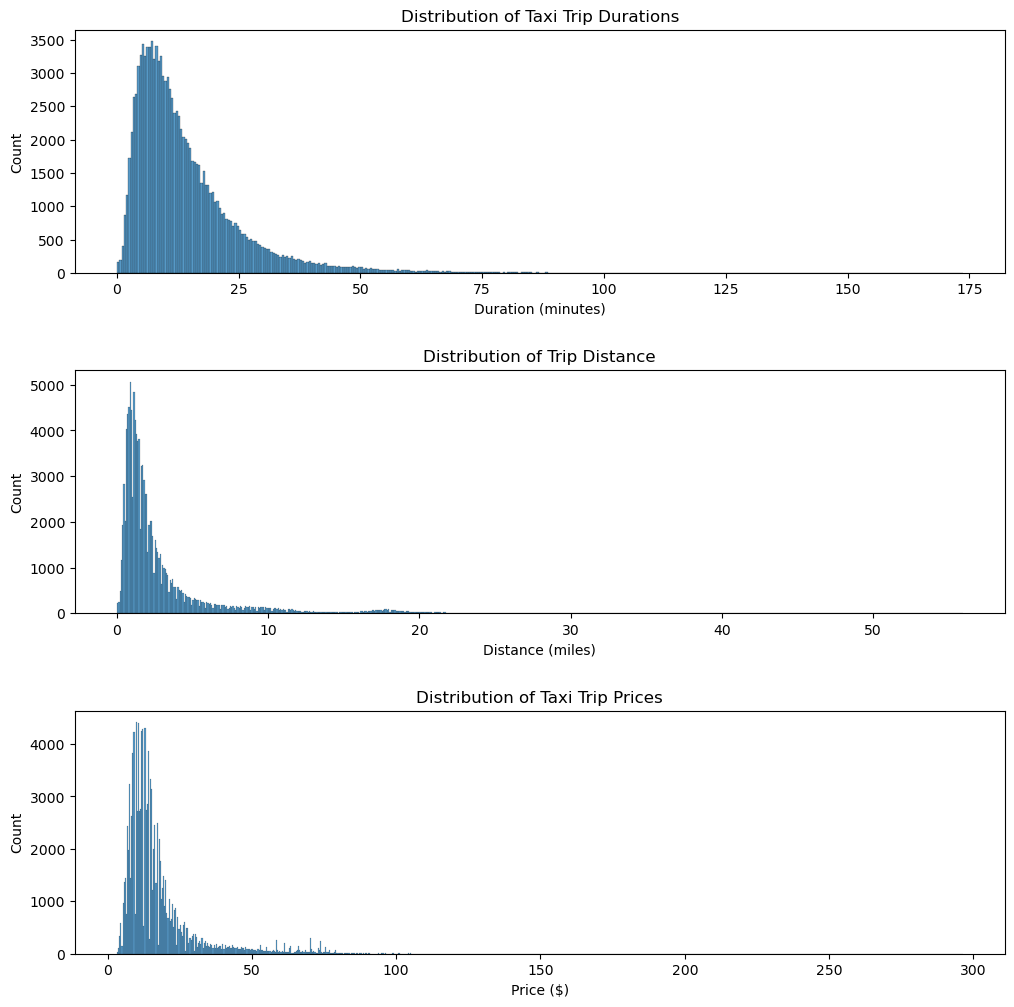### 4.b. Taxi Trip Pricing

The code and graph below show the composition of a taxi trip price. We only include standard-rate trips to show the percentage of tips in total price, as our dataset only has the tip_amount column for trips paid with credit card.

```from pyspark.sql.functions import mean, sum

avg_prices = df.where(col('payment_type')=='1').select(
mean("fare_amount").alias("avg_fare"),
mean("tip_amount").alias("avg_tips"),
mean("extra").alias("avg_extra"),
mean("mta_tax").alias("avg_tax"),
mean("tolls_amount").alias("avg_tolls"),
mean("imp_surcharge").alias("avg_surcharge")
)
```
```avg_prices = avg_prices.withColumn('avg_total', col('avg_fare')+ col('avg_extra')+ col('avg_tips')+ col('avg_tax')+ col('avg_surcharge')+ col('avg_tolls'))
avg = avg_prices.first()
```
```
```
```fare_pctg = avg_prices.select(col('avg_fare')/col('avg_total')*100).collect()
tips_pctg = avg_prices.select(col('avg_tips')/col('avg_total')*100).collect()
extra_pctg = avg_prices.select(col('avg_extra')/col('avg_total')*100).collect()
tax_pctg = avg_prices.select(col('avg_tax')/col('avg_total')*100).collect()
tolls_pctg = avg_prices.select(col('avg_tolls')/col('avg_total')*100).collect()
surcharge_pctg = avg_prices.select(col('avg_surcharge')/col('avg_total')*100).collect()
```
```
```
```import matplotlib.pyplot as plt
labels = ['Fare', 'Tips', 'Extra', 'Tax', 'Tolls', 'Surcharge']
sizes = [fare_pctg, tips_pctg, extra_pctg,  tax_pctg, tolls_pctg, surcharge_pctg]
plt.pie(sizes, labels=labels, autopct='%1.1f%%')
plt.axis('equal')
plt.title('Average Percentage of Price Components')
plt.show()
```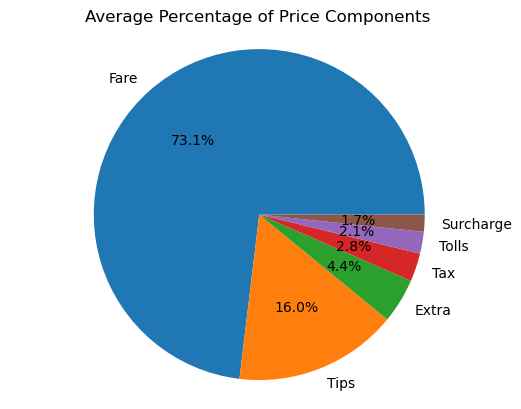This pie chart indicates that the majority of taxi trip price comes from distance-and-time calculated fare, accounting for 73% of the total price. An average of 16% of total price is attributed to tips. Fees, tax, and surcharges account for the rest of total price.

```df= spark.read.format("parquet").load("gs://is843-team6/notebooks/jupyter/df.parquet")
```

## 5. Exploratory Data Analysis

### 5.a. Demand

Revenue = Demand * Price
According to the equation above, we want to start with looking at the demand patterns for NYC taxi trips.

#### Question 1: How does the distribution of taxi demand differ across the days of the week, time of day, and month?

##### Taxi demand distribution across hours of a day in 2021
```df.createOrReplaceTempView('df')
```
```from pyspark.sql.functions import col
df_2021 = df.where((col('year')==2021)&(col('pickup_ET')!='2121-11-07')&(col('pickup_ET')!='2021-03-14'))
```
```df_2021.createOrReplaceTempView('df_2021')
```
```hourly_demand2021 = spark.sql("""
SELECT hour, COUNT(*) AS demand_hour
FROM df_2021
GROUP BY hour
ORDER BY hour
""")

hourly_demand2021_pd = hourly_demand2021.toPandas()
```
```
```
```sns.set_style('whitegrid')
plt.figure(figsize=(6,6))
plot = sns.catplot(x='hour', y='demand_hour',data=hourly_demand2021_pd,kind='bar',color='red')
plot.set(xlabel='hour of day', ylabel='average taxi demand')
plt.title('Average Taxi Demand of a Day for 2021')
plot.set_xticklabels(rotation=90)
plt.show()
```
`<Figure size 600x600 with 0 Axes>`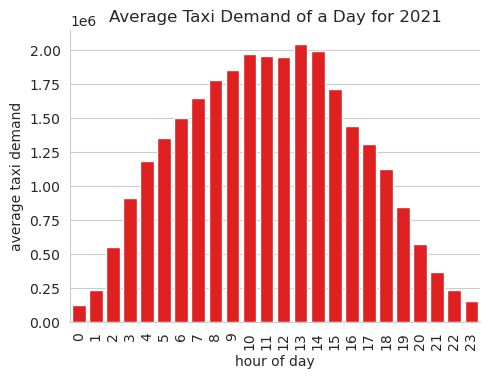Rush hours are 10 am to 3 pm, when the demand for taxis is high. For drivers to optimize their income, avoiding rush hours that may see traffic congestion and midnight when taxi demand is too low would be a good strategy.

##### Taxi demand distribution across days of the week
```df_weekly_demand_pd = df_weekly_demand.toPandas()
```
```
```
```sns.set_style('whitegrid')
plt.figure(figsize=(8,6))
plot = sns.catplot(x='day_of_week', y='avg_demand',data=df_weekly_demand_pd,kind='bar',color='red',order=['Monday','Tuesday','Wednesday','Thursday','Friday','Saturday','Sunday'])
plot.set(xlabel='day of the week', ylabel='average taxi demand')
plt.title('Distribution of Average Taxi Demand over a Week')
plot.set_xticklabels(rotation=45)
plt.show()
```
`<Figure size 800x600 with 0 Axes>`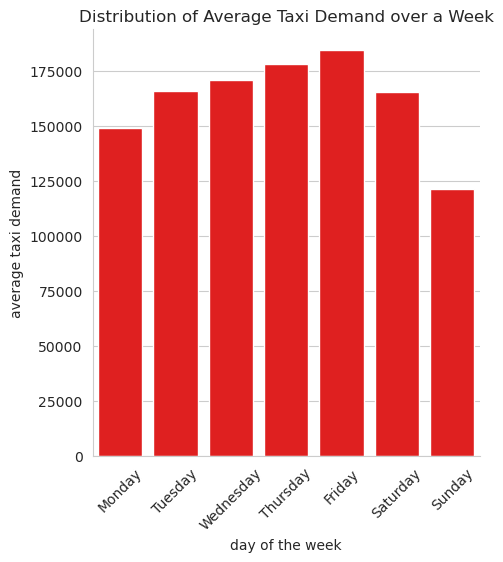Based on the graph above, from 2018 to 2021, Sunday saw the lowest demand for taxi trips, while Friday saw the highest demand. If drivers want to make more money, they may not take business on these two days because Friday may see congestion, and you may only take a few customers on Sunday.

##### Hourly taxi demand distribution

Count the number of taxi trips for each hour of a day

```hourly_distribution = df.groupBy("hour").count().orderBy("hour")
hourly_distribution.show(25)
```
```[Stage 105:====================================================>(229 + 1) / 230]
```
```+----+--------+
|hour|   count|
+----+--------+
|   0| 1851309|
|   1| 3128851|
|   2| 6334319|
|   3| 9349140|
|   4|10813174|
|   5|11137490|
|   6|11521145|
|   7|12229850|
|   8|12805310|
|   9|13202922|
|  10|13714879|
|  11|13308316|
|  12|13422739|
|  13|14877029|
|  14|15236563|
|  15|14033836|
|  16|12990995|
|  17|12459798|
|  18|10918785|
|  19| 8513946|
|  20| 5990693|
|  21| 4125097|
|  22| 2903280|
|  23| 2068214|
+----+--------+

```
```
```
##### Monthly taxi demand distribution in 2019, 2020, and 2021
```from pyspark.sql.functions import year, month, count, date_trunc
import seaborn as sns

df_filtered = df.filter((df['year'] == 2019) |
(df['year'] == 2020) |
(df['year'] == 2021))
```
```df_monthly_distribution = df_filtered.groupBy(("year"),
month("pickup_ET").alias("month")) \
.count() \
.orderBy("year", "month")
```
``` df_monthly_distribution_graph= sns.FacetGrid(df_monthly_distribution.toPandas(), col="year", col_wrap=3, sharey=False)
df_monthly_distribution_graph.map(sns.barplot, "month", "count", palette=["#ff4c4c"])
df_monthly_distribution_graph.set_axis_labels("Month", "Count of Trips")
```
```/opt/conda/miniconda3/lib/python3.8/site-packages/seaborn/axisgrid.py:670: UserWarning: Using the barplot function without specifying `order` is likely to produce an incorrect plot.
warnings.warn(warning)
```
`<seaborn.axisgrid.FacetGrid at 0x7f5f2696b700>`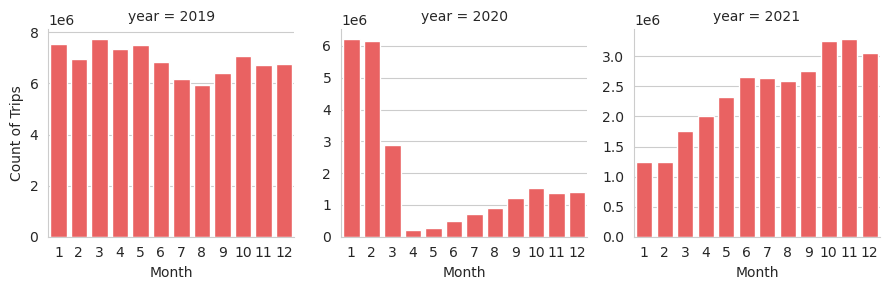Based on the graph above, it is evident that in 2019 most months had a similar distribution of trips, with winter months showing slightly higher amounts when compared to summer. January and February of 2020 displayed nearly identical results. However, there was a significant decrease in taxi trips starting in March due to the covid pandemic and stay-at-home mandates. Consequently, the entirety of 2020 displayed much lower numbers than the previous years. However, in 2021, with the distribution of the covid vaccine and the start of a return to normalcy, the number of trips shows an upward trend.

### Question 2: How does taxi demand differ across different time categories?

```'''
* Late Night: This is the period between midnight and sunrise, usually from 12:00 AM to 5:59 AM.

* Morning: This is the period after sunrise and before noon, usually from 6:00 AM to 11:59 AM.

* Afternoon: This is the period between noon and evening, usually from 12:00 PM to 4:59 PM.

* Evening: This is the period between late afternoon and late night, usually from 5:00 PM to 8:59 PM.

* Night: This is the period between late evening and early morning, usually from 9:00 PM to 11:59 PM.'''

from pyspark.sql.functions import hour, when

# Categorizing the pickup_ET time in different time categories (5 in our case)
spark_df_changed_casted_dataframe_f = df.withColumn('pickup_time_category', \
when((df.hour >= 0) & (df.hour <= 5), 'Late Night') \
.when((df.hour >= 6) & (df.hour <= 11), 'Morning') \
.when((df.hour >= 12) & (df.hour <= 16), 'Afternoon') \
.when((df.hour >= 17) & (df.hour <= 20), 'Evening') \
.otherwise('Night'))

# Show the resulting dataframe
spark_df_changed_casted_dataframe_f.select('pickup_ET', 'pickup_time_category').show(5)
```
```+-------------------+--------------------+
|          pickup_ET|pickup_time_category|
+-------------------+--------------------+
|2020-12-31 19:43:30|             Evening|
|2020-12-31 19:15:48|             Evening|
|2020-12-31 19:19:57|             Evening|
|2020-12-31 19:44:58|             Evening|
|2020-12-31 19:06:11|             Evening|
+-------------------+--------------------+
only showing top 5 rows

```
```spark_df_changed_casted_dataframe_f.cache()
```
`DataFrame[vendor_id: string, pickup_datetime: timestamp, dropoff_datetime: timestamp, passenger_count: bigint, trip_distance: decimal(38,9), rate_code: string, store_and_fwd_flag: string, payment_type: string, fare_amount: decimal(38,9), extra: decimal(38,9), mta_tax: decimal(38,9), tip_amount: decimal(38,9), tolls_amount: decimal(38,9), imp_surcharge: decimal(38,9), airport_fee: decimal(38,9), total_amount: decimal(38,9), pickup_location_id: string, dropoff_location_id: string, year: int, duration: double, pickup_ET: timestamp, dropoff_ET: timestamp, day_of_week: string, hour: int, pickup_time_category: string]`
```import matplotlib.pyplot as plt
from pyspark.sql.functions import count

# Calculating demand of number of rides through count by pickup time category
pickup_demand = spark_df_changed_casted_dataframe_f.groupBy('pickup_time_category').agg(count('*').alias('demand')).orderBy('demand', ascending=False)

# Displaying pickup demand in decreasing order
print("Pickup Demand:")
pickup_demand.show()
```
```Pickup Demand:
```
```[Stage 117:====================================================>(227 + 3) / 230]
```
```+--------------------+--------+
|pickup_time_category|  demand|
+--------------------+--------+
|             Morning|76782422|
|           Afternoon|70561162|
|          Late Night|42614283|
|             Evening|37883222|
|               Night| 9096591|
+--------------------+--------+

```
```
```
```pickup_demand = spark_df_changed_casted_dataframe_f.groupBy('pickup_time_category').agg(count('*').alias('demand')).orderBy('demand', ascending=False)

# Plot pie chart of demand by pickup time category
plt.pie(pickup_demand.select('demand').rdd.flatMap(lambda x: x).collect(), labels=pickup_demand.select('pickup_time_category').rdd.flatMap(lambda x: x).collect(), autopct='%1.1f%%', startangle=90)
plt.axis('equal')
plt.title('Demand for different time categories (pickup)')
plt.show()
```
```
```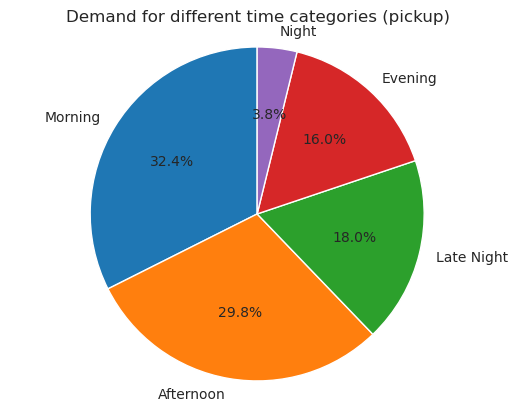• Most of the Taxis were taken in Morning and Afternoon Time
• Least Taxis were taken at night from: 9:00 PM to 11:59 PM.

### Question 3: What are the ten most frequently traveled routes for taxi rides in NYC?

Building on our findings about demand for different times, we want to look into locations and routes that lead to high revenue.

```B = spark.read.format("bigquery").option("table", "bigquery-public-data.new_york_taxi_trips.taxi_zone_geom").load().select("zone_name", "zone_id")
A_with_zone = df.join(B, df.dropoff_location_id == B.zone_id, how="left").withColumn("dropoff_zone_name", B.zone_name)\
.drop("zone_id", "zone_name").join(B, df.pickup_location_id == B.zone_id, how="left").withColumn("pickup_zone_name", B.zone_name).drop("zone_id", "zone_name")
```
```A_with_zone.show(5)
```
```23/04/30 23:19:26 WARN org.apache.spark.sql.catalyst.util.package: Truncated the string representation of a plan since it was too large. This behavior can be adjusted by setting 'spark.sql.debug.maxToStringFields'.
[Stage 137:>                                                        (0 + 1) / 1]
```
```+---------+-------------------+-------------------+---------------+-------------+---------+------------------+------------+------------+-----------+-----------+-----------+------------+-------------+--------------+------------+------------------+-------------------+----+--------+-------------------+-------------------+-----------+----+--------------------+-----------------+
|vendor_id|    pickup_datetime|   dropoff_datetime|passenger_count|trip_distance|rate_code|store_and_fwd_flag|payment_type| fare_amount|      extra|    mta_tax| tip_amount|tolls_amount|imp_surcharge|   airport_fee|total_amount|pickup_location_id|dropoff_location_id|year|duration|          pickup_ET|         dropoff_ET|day_of_week|hour|   dropoff_zone_name| pickup_zone_name|
+---------+-------------------+-------------------+---------------+-------------+---------+------------------+------------+------------+-----------+-----------+-----------+------------+-------------+--------------+------------+------------------+-------------------+----+--------+-------------------+-------------------+-----------+----+--------------------+-----------------+
|        1|2021-01-01 00:43:30|2021-01-01 01:11:06|              1| 14.700000000|        1|                 N|           1|42.000000000|0.500000000|0.500000000|8.650000000|        0E-9|  0.300000000|-999.000000000|51.950000000|               132|                165|2021|    27.6|2020-12-31 19:43:30|2020-12-31 20:11:06|   Thursday|  19|             Midwood|      JFK Airport|
|        1|2021-01-01 00:15:48|2021-01-01 00:31:01|              0| 10.600000000|        1|                 N|           1|29.000000000|0.500000000|0.500000000|6.050000000|        0E-9|  0.300000000|-999.000000000|36.350000000|               138|                132|2021|   15.22|2020-12-31 19:15:48|2020-12-31 19:31:01|   Thursday|  19|         JFK Airport|LaGuardia Airport|
|        2|2021-01-01 00:19:57|2021-01-01 00:43:03|              3| 10.740000000|        1|                 N|           1|32.500000000|0.500000000|0.500000000|4.000000000|        0E-9|  0.300000000|-999.000000000|40.300000000|               264|                231|2021|    23.1|2020-12-31 19:19:57|2020-12-31 19:43:03|   Thursday|  19|TriBeCa/Civic Center|             null|
|        2|2021-01-01 00:44:58|2021-01-01 01:07:41|              2|  5.850000000|        1|                 N|           1|21.500000000|0.500000000|0.500000000|5.060000000|        0E-9|  0.300000000|-999.000000000|30.360000000|               249|                238|2021|   22.72|2020-12-31 19:44:58|2020-12-31 20:07:41|   Thursday|  19|Upper West Side N...|     West Village|
|        2|2021-01-01 00:06:11|2021-01-01 00:23:40|              1|  8.060000000|        1|                 N|           1|24.500000000|0.500000000|0.500000000|5.660000000|        0E-9|  0.300000000|-999.000000000|33.960000000|                75|                264|2021|   17.48|2020-12-31 19:06:11|2020-12-31 19:23:40|   Thursday|  19|                null|East Harlem South|
+---------+-------------------+-------------------+---------------+-------------+---------+------------------+------------+------------+-----------+-----------+-----------+------------+-------------+--------------+------------+------------------+-------------------+----+--------+-------------------+-------------------+-----------+----+--------------------+-----------------+
only showing top 5 rows

```
```
```
```from pyspark.sql.functions import count, avg, expr
from pyspark.sql.window import Window

df_zone = A_with_zone.select("pickup_zone_name", "dropoff_zone_name", "fare_amount", "pickup_datetime", "dropoff_datetime", "trip_distance") \
.filter((A_with_zone.pickup_zone_name.isNotNull()) & (A_with_zone.dropoff_zone_name.isNotNull()) & (A_with_zone.fare_amount.isNotNull()) & (A_with_zone.pickup_datetime.isNotNull()) & (A_with_zone.dropoff_datetime.isNotNull()) & (A_with_zone.trip_distance.isNotNull()))
#new column
df_zone = df_zone.withColumn("duration_minutes", expr("(UNIX_TIMESTAMP(dropoff_datetime) - UNIX_TIMESTAMP(pickup_datetime))/60"))
#groupby
df_zone = df_zone.groupBy("pickup_zone_name", "dropoff_zone_name") \
.agg(count("*").alias("trip_count"),
avg("fare_amount").alias("avg_fare_amount"),
avg("duration_minutes").alias("avg_duration_minutes"),
avg("trip_distance").alias("avg_trip_distance")) \
.orderBy("trip_count", ascending=False) \
.limit(10)

df_zone.show()
```
```[Stage 141:========================================>                (5 + 2) / 7]
```
```+--------------------+--------------------+----------+---------------+--------------------+-----------------+
|    pickup_zone_name|   dropoff_zone_name|trip_count|avg_fare_amount|avg_duration_minutes|avg_trip_distance|
+--------------------+--------------------+----------+---------------+--------------------+-----------------+
|Upper East Side S...|Upper East Side N...|   1536223|6.4637527170000|   6.567530657983904|  1.0572492410000|
|Upper East Side N...|Upper East Side S...|   1301958|7.0365252410000|   7.903893942815366|  1.0562288340000|
|Upper East Side N...|Upper East Side N...|   1230563|5.1022814760000|   4.508901779104363|  0.6337434900000|
|Upper East Side S...|Upper East Side S...|   1166238|5.4694903610000|   5.212215145336262|  0.6620207370000|
|Upper West Side S...|Upper West Side N...|    687418|5.4631325630000|    4.91102594539761|  0.8376672270000|
|Upper West Side S...| Lincoln Square East|    667270|5.8577696130000|   5.711464999175746|  0.8722473960000|
| Lincoln Square East|Upper West Side S...|    630715|6.1414540010000|    6.07700062627336|  0.9803069220000|
|Upper East Side S...|        Midtown East|    626600|6.9054814870000|  7.9227933822747065|  0.9700193270000|
|Upper East Side S...|      Midtown Center|    610173|7.9622868920000|   9.977792090658436|  1.0930640490000|
|Upper West Side N...|Upper West Side S...|    596473|5.4437153570000|   4.925779764269852|  0.8031172580000|
+--------------------+--------------------+----------+---------------+--------------------+-----------------+

```
```
```
```import matplotlib.pyplot as plt
import pandas as pd
pandas_df = df_zone.toPandas()
pandas_df = pandas_df.set_index(["pickup_zone_name", "dropoff_zone_name"])
pandas_df.plot(kind="bar", y="trip_count")
plt.title("Top 10 Taxi Routes in NYC in 2022")
plt.xlabel("Pickup-Dropoff Location")
plt.ylabel("Number of Trips")
plt.show()
```
```
```